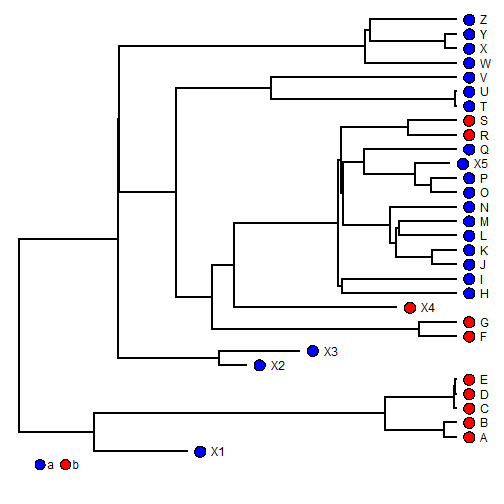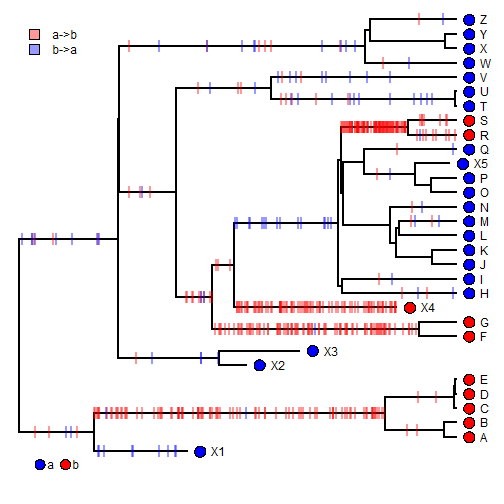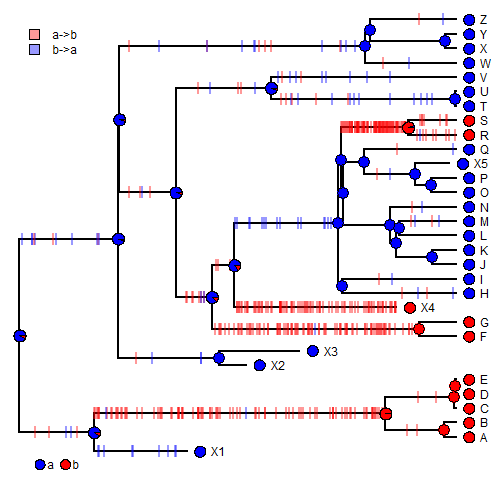## Tuesday, May 17, 2016

### Using `markChanges` to show the distribution of implied changes on a tree from stochastic mapping

phytools has a function called `markChanges` that can be used to highly changes of different types on the tree. Various uses of this function are documented here; however they focus on marking the changes from a single stochastic map history. Alternatively, we might like to look at the distribution of changes across a sample of stochastic maps.

This turns out to be really easy to do.

``````library(phytools)
tree
``````
``````##
## Phylogenetic tree with 31 tips and 30 internal nodes.
##
## Tip labels:
##  X1, A, B, C, D, E, ...
##
## Rooted; includes branch lengths.
``````
``````x
``````
``````##  X1   A   B   C   D   E  X2  X3   F   G  X4   H   I   J   K   L   M   N
## "a" "b" "b" "b" "b" "b" "a" "a" "b" "b" "b" "a" "a" "a" "a" "a" "a" "a"
##   O   P  X5   Q   R   S   T   U   V   W   X   Y   Z
## "a" "a" "a" "a" "b" "b" "a" "a" "a" "a" "a" "a" "a"
``````
``````colors<-setNames(c("blue","red"),c("a","b"))
dotTree(tree,x,colors=colors)
``````We can do stochastic mapping as follows:

``````## first let's pick a model
fitER<-fitMk(tree,x,model="ER")
fitER
``````
``````## Object of class "fitMk".
##
## Fitted (or set) value of Q:
##           a         b
## a -0.027553  0.027553
## b  0.027553 -0.027553
##
## Fitted (or set) value of pi:
##   a   b
## 0.5 0.5
##
## Log-likelihood: -10.956725
``````
``````fitARD<-fitMk(tree,x,model="ER")
fitARD
``````
``````## Object of class "fitMk".
##
## Fitted (or set) value of Q:
##           a         b
## a -0.027553  0.027553
## b  0.027553 -0.027553
##
## Fitted (or set) value of pi:
##   a   b
## 0.5 0.5
##
## Log-likelihood: -10.956725
``````
``````LR<--2*(logLik(fitER)-logLik(fitARD))
P<-pchisq(LR,df=1,lower.tail=FALSE)
P ## we cannot reject "ER"
``````
``````##  1
``````
``````trees<-make.simmap(tree,x,nsim=100,model="ER")
``````
``````## make.simmap is sampling character histories conditioned on the transition matrix
##
## Q =
##             a           b
## a -0.02755304  0.02755304
## b  0.02755304 -0.02755304
## (estimated using likelihood);
## and (mean) root node prior probabilities
## pi =
##   a   b
## 0.5 0.5
``````
``````## Done.
``````
``````trees
``````
``````## 100 phylogenetic trees with mapped discrete characters
``````

Now, let's make our plot. Unlike in the example referenced above, here I'm going to overlay the changes on on a `dotTree` plot, instead of on any single stochastic map. To make the density of the marked changes a little bit easier to read, I'm going to make them semi-transparent with the aide of the phytools internal function `make.transparent` (which I borrowed from something Josef Uyeda shared with me a long time ago):

``````dotTree(tree,x,colors=colors)
mt<-phytools:::make.transparent ## using the internal function
nulo<-sapply(trees,markChanges,sapply(colors,mt,0.4))
mt,0.4),prompt=FALSE,x=0.5,y=30,vertical=TRUE)
``````If you wanted to go crazy, you could also add the posterior probabilities at nodes as follows:

``````obj<-summary(trees)
obj
``````
``````## 100 trees with a mapped discrete character with states:
##  a, b
##
## trees have 5.1 changes between states on average
##
## changes are of the following types:
##       a,b  b,a
## x->y 4.25 0.85
##
## mean total time spent in each state is:
##                a          b    total
## raw  141.2496391 39.5391057 180.7887
## prop   0.7812966  0.2187034   1.0000
``````
``````dotTree(tree,x,colors=colors)
nulo<-sapply(trees,markChanges,sapply(colors,mt,0.4))
mt,0.4),prompt=FALSE,x=0.5,y=30,vertical=TRUE)
nodelabels(pie=obj\$ace,cex=0.6,piecol=colors)
``````Although that could be a bit excessive.

In any case, it gives a good idea of where on the tree changes in state most likely occured. For instance, it is very interesting to note that changes `a->b` most likely occurred independently on the lineages leading to `X4` and `(G,F)`, rather than once in their common ancestor followed by a reversion `b->a`.

That's all.